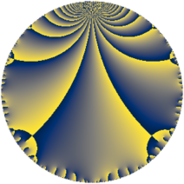# Properties

 Label 135.4.aLevel $135$ Weight $4$ Character orbit 135.a Rep. character $\chi_{135}(1,\cdot)$ Character field $\Q$ Dimension $16$ Newform subspaces $8$ Sturm bound $72$ Trace bound $2$

# Related objects

## Defining parameters

 Level: $$N$$ $$=$$ $$135 = 3^{3} \cdot 5$$ Weight: $$k$$ $$=$$ $$4$$ Character orbit: $$[\chi]$$ $$=$$ 135.a (trivial) Character field: $$\Q$$ Newform subspaces: $$8$$ Sturm bound: $$72$$ Trace bound: $$2$$ Distinguishing $$T_p$$: $$2$$

## Dimensions

The following table gives the dimensions of various subspaces of $$M_{4}(\Gamma_0(135))$$.

Total New Old
Modular forms 60 16 44
Cusp forms 48 16 32
Eisenstein series 12 0 12

The following table gives the dimensions of the cuspidal new subspaces with specified eigenvalues for the Atkin-Lehner operators and the Fricke involution.

$$3$$$$5$$FrickeDim.
$$+$$$$+$$$$+$$$$4$$
$$+$$$$-$$$$-$$$$2$$
$$-$$$$+$$$$-$$$$4$$
$$-$$$$-$$$$+$$$$6$$
Plus space$$+$$$$10$$
Minus space$$-$$$$6$$

## Trace form

 $$16 q + 58 q^{4} + 68 q^{7} + O(q^{10})$$ $$16 q + 58 q^{4} + 68 q^{7} + 30 q^{10} - 100 q^{13} + 754 q^{16} - 64 q^{19} - 348 q^{22} + 400 q^{25} + 236 q^{28} + 296 q^{31} + 570 q^{34} - 412 q^{37} + 480 q^{40} - 736 q^{43} - 3090 q^{46} - 540 q^{49} - 772 q^{52} + 60 q^{55} - 3744 q^{58} + 668 q^{61} + 4600 q^{64} + 4244 q^{67} - 540 q^{70} + 788 q^{73} - 3814 q^{76} + 716 q^{79} - 2556 q^{82} + 120 q^{85} - 10212 q^{88} - 2084 q^{91} + 4116 q^{94} + 3212 q^{97} + O(q^{100})$$

## Decomposition of $$S_{4}^{\mathrm{new}}(\Gamma_0(135))$$ into newform subspaces

Label Dim $A$ Field CM Traces A-L signs $q$-expansion
$a_{2}$ $a_{3}$ $a_{5}$ $a_{7}$ 3 5
135.4.a.a $1$ $7.965$ $$\Q$$ None $$-2$$ $$0$$ $$5$$ $$0$$ $+$ $-$ $$q-2q^{2}-4q^{4}+5q^{5}+24q^{8}-10q^{10}+\cdots$$
135.4.a.b $1$ $7.965$ $$\Q$$ None $$-1$$ $$0$$ $$-5$$ $$-6$$ $+$ $+$ $$q-q^{2}-7q^{4}-5q^{5}-6q^{7}+15q^{8}+\cdots$$
135.4.a.c $1$ $7.965$ $$\Q$$ None $$1$$ $$0$$ $$5$$ $$-6$$ $+$ $-$ $$q+q^{2}-7q^{4}+5q^{5}-6q^{7}-15q^{8}+\cdots$$
135.4.a.d $1$ $7.965$ $$\Q$$ None $$2$$ $$0$$ $$-5$$ $$0$$ $-$ $+$ $$q+2q^{2}-4q^{4}-5q^{5}-24q^{8}-10q^{10}+\cdots$$
135.4.a.e $3$ $7.965$ 3.3.1772.1 None $$-5$$ $$0$$ $$-15$$ $$-4$$ $-$ $+$ $$q+(-2+\beta _{1})q^{2}+(7-3\beta _{1}+\beta _{2})q^{4}+\cdots$$
135.4.a.f $3$ $7.965$ 3.3.5637.1 None $$-1$$ $$0$$ $$15$$ $$44$$ $-$ $-$ $$q-\beta _{1}q^{2}+(7+\beta _{1}+\beta _{2})q^{4}+5q^{5}+\cdots$$
135.4.a.g $3$ $7.965$ 3.3.5637.1 None $$1$$ $$0$$ $$-15$$ $$44$$ $+$ $+$ $$q+\beta _{1}q^{2}+(7+\beta _{1}+\beta _{2})q^{4}-5q^{5}+\cdots$$
135.4.a.h $3$ $7.965$ 3.3.1772.1 None $$5$$ $$0$$ $$15$$ $$-4$$ $-$ $-$ $$q+(2-\beta _{1})q^{2}+(7-3\beta _{1}+\beta _{2})q^{4}+5q^{5}+\cdots$$

## Decomposition of $$S_{4}^{\mathrm{old}}(\Gamma_0(135))$$ into lower level spaces

$$S_{4}^{\mathrm{old}}(\Gamma_0(135)) \cong$$ $$S_{4}^{\mathrm{new}}(\Gamma_0(5))$$$$^{\oplus 4}$$$$\oplus$$$$S_{4}^{\mathrm{new}}(\Gamma_0(9))$$$$^{\oplus 4}$$$$\oplus$$$$S_{4}^{\mathrm{new}}(\Gamma_0(15))$$$$^{\oplus 3}$$$$\oplus$$$$S_{4}^{\mathrm{new}}(\Gamma_0(27))$$$$^{\oplus 2}$$$$\oplus$$$$S_{4}^{\mathrm{new}}(\Gamma_0(45))$$$$^{\oplus 2}$$